# 2020.9 月赛题解

Once edited 2 年，5 月前

### 花絮

• 久违的六个题月赛。
• 全场几乎没人提问，是否侧面反映出题目细致程度有所提高（出题组先自恋五秒）。
# Tag Idea Developer Tester
C 贪心 构造 动态规划 cs2017 Xiejiadong Once bingoier 10185101232
D 计数 容斥原理 changtiaoraplanqiu changtiaoraplanqiu Once 10185101232
E 数论 构造 Xiejiadong bingoier 10185101232
F Trie 树套树 Xiejiadong Once bingoier NULL

### Problem A 击鼓传花

##### First Solved: Yewenw

• 当 $l=0,f(a,b,l,r)=0$
• 当 $\left \lceil \frac{l}{a} \right \rceil \le \left \lfloor \frac{r}{a} \right \rfloor$ 则 $\exists \left \lceil \frac{l}{a} \right \rceil \le \left \lfloor \frac{r}{a} \right \rfloor$ 存在最小的 $x_0=\left \lceil \frac{l}{a} \right \rceil$, 使得 $l\le ax_0\le r$ ，显然 $f(a,b,l,r)=x_0$
• 当 $\left \lceil \frac{l}{a} \right \rceil > \left \lfloor \frac{r}{a} \right \rfloor$ ，存在 $M\in N ^ *$ 使得 $aM < l \le r < a(M+1)$ $$aM < l \le ax-bk\le r < a(M+1) \Leftrightarrow -a(M+1) < -r \le bk-ax\le -l < -aM\Leftrightarrow a(x-M-1)\le ax-r < bk\le ax-l\le a(x-M)$$

### Problem D 礼物箱

##### First Solved: paleprince

min-max 容斥.

$$Y = \max_{i=1,2,\dots,n}(X_{i})$$

$$\max_{i=1,2,\dots,n}(X_{i}) = \sum_{i}X_{i} - \sum_{i < j}min(X_{i},X_j) + \dots + (-1)^{n+1}\min_{i=1,2,\dots,n}(X_i)$$

$$\frac{\binom{m}{1}}{\binom{n+m}{1}}\frac{n}{n+m-1} + 2\frac{\binom{m}{2}}{\binom{n+m}{2}}\frac{n}{n+m-2} + 3\frac{\binom{m}{3}}{\binom{n+m}{3}}\frac{n}{n+m-3} + \dots + m\frac{1}{\binom{n+m}{m}}\frac{n}{n+m-m} \$$

$$dp[n][m] = \frac{m}{m+n}(dp[n][m-1]+1) + \frac{n}{m+n}$$

### Problem E 加密的情书

##### First Solved: SuperSodaSea

• 若 $x=0$，则 $f(x)=0$
• 若 $x\neq 0, x\mod 9\neq 0$，则 $f(x)=x\mod 9$
• 若 $x\neq 0, x\mod 9=0$，则 $f(x)=9$

Once ：为了增加签到难度，bingoier 在输入范围中加入了 $0$，不要忘记特判。题面强调自然数的概念也是希望大家能意识到 $0$ 的特殊性。

Once ：这六道题题面是我写的，大家有喜欢的不喜欢的欢迎吐槽。

### Problem F 动态树

#### 解法 2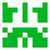D 题题解的对期望的容斥我没太看懂，看样子复杂度应该是$\, O(2^n + m^2) \,$的。不过我写的复杂度大概是$\, O(nm^2) \,$的。就是分别计算拿到的最后一种礼物是$\, i \,$的期望，然后加起来。计算的时候只需要让另外$\, n - 1 \,$种至少选了$\, 1 \,$个，这里用类似背包的方法就行了。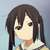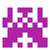E题 n=1 且唯一的密文为0时 为什么不考虑l==r==0的情况?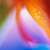C 不用 DP，直接把一段正负 1 交替的子段合并到只有 1 或者 -1 似乎也行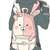D 邦邦，出题人头像好评（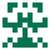F题的第二种方法，“我们在 Trie 上找对应结点的时候，我们需要保证走到的 Trie 结点的子树中包含至少一个在 对应子树编号区间内的 01 串即可“，这个要怎么维护呢？